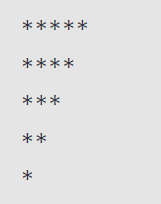Related Tags

triangle pattern
communitycreator

# Javascript downward triangle pattern

Ayush Trivedi

### Overview

Star Pattern is an exciting programming problem to do. It tests your knowledge of using language concepts, mainly loops and recursion. This problem needs creative thinking.

There are several patterns problems to solve, but in this shot, we learn to print a downward triangle star (*) pattern with the help of Javascript. The downward triangle pattern is similar to the left triangle (right-angled from the left side) in a downward direction.

Below is the shape which we will print using * in the console. We have to use nested for loops. The outer loop will be for the row (height) of the triangle, and the nested loop will be responsible for the column.As you can see in the image, stars are decreasing from n to 1, where n is the number of rows that is the triangle’s height. The logic for this is simple, you have to iterate the outer loop for n times, whereas the inner loop will iterate from 0 times decreasing to n-i for each outer loop.

let n = 5;

for (let i = 0; i < n; i++) {
// printing star
for (let k = 0; k < n - i; k++) {
process.stdout.write('*');
}
console.log();
}

Click on the run button and check out the output. You can change the value of the row and get the triangle of the height of your choice.

### Code explanation

• In line 1, we initialize a number that is the height of the triangle or number of rows.

• In line 3, we have our outer for loop, which will iterate over the rows of the triangle (that is 5 here).

• In line 5, in this inner nested for loop, which will iterate for k=0 to k > n-i, i is the outer loop number.

• In line 6, the standard method of javascript is used to print the star (*). process.stdout.write() will print the star (*).

• The number of times the nested for loop runs is equal to the number of stars it prints.

• In line 7, we use console.log() with null as it will change to a new line.

• We can use process.stdout.write('\n') to change the line.

### Result

We have successfully completed the task to print stars in the form that looks like a downward triangle.

RELATED TAGS

triangle pattern
communitycreator

CONTRIBUTOR

Ayush Trivedi
RELATED COURSES

View all Courses

Keep Exploring

Learn in-demand tech skills in half the time